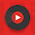## Solved Objective Questions from Basic Electrical Engineering

 At resonance, the RLC series circuit behaves as a
A. Purely resistive circuit
B. Purely inductive circuit
C. Capacitive circuit
D. Reactive Circuit

Answer: A
 The demand factor for the electrical system is the ratio of
A. Maximum demand to connected load
B. Maximum demand to average load
C. Average power to maximum power
D. Relative power to total power

Answer: A
 When a low resistance is connected in parallel with a high resistance, the combined resistance is
A. Always more than the high resistance
B. Always less than the low resistance
C. Always between the high resistance & low resistance
D. Either lower of higher than low resistance depending on the value of high resistance

Answer: B
 In RLC circuit, the phase of current with respect to the circuit voltage will be
A. Leading
B. Same
C. Lagging
D. Depends on L & C values

Answer: D
 The superposition theorem is based on the concept of
A. Duality
B. Linearity
C. Reciprocity
D. Non - Linearity

Answer: B
 Which of the following is same is all part of the series circuit?
A. Voltage
B. Current
C. Power
D. Resistance

Answer = B
 An electric machine will have high efficiency when
A. Input/output ratio is low
B. Reactive power is more
C. KWh consumption is low
D. Losses are low

Answer: D
 In a PNP circuit, the collector
A. Has a arrow pointing inward
B. Is positive with respect to the emitter
C. Is biased at a small fraction of the base bias
D. Is negative with respect to the emitter

Answer: D
 A PNP transistor can be replaced with an NPN device and the circuit will do the same thing, provided that
A. The power supply or battery polarity is reversed
B. The collector and emitter leads are interchanged
C. The arrow is pointing inward
D. A PNP transistor can never be replaced with NPN transistor

Answer: A
 The form factor of a ac voltage is
A.  RMS value/ Average value
B.  Average Value/ RMS Value
C.  Instantaneous Value/ Average value
D.  Average value/ Instantaneous value

Answer: A
5:56 PM

#### 4 comments:

1.You have posted a good preparation material for electrical engineers, I like it..

2.thanks for your comments

1.SIR U R WORKING AWESOME PLZ UPLOAD MORE QUESTIONS AS SOON AS POSSIBLEEEEEEEEE

3.bimal* 06..08.2014August 6, 2014 at 2:08 AM

Reall good question thanks you sir........